﻿ Boffin Access Limited - Open Access Journals - Scholarly Publishing-STEM# ADVANCES IN COMPUTER SCIENCES (ISSN:2517-5718)

### Alexander Yurkin*

Independant Researcher, Puschino, Moscow, Russian Federation

### CitationCitation COPIED

Yurkin A. New Analogues of the Pascal Triangle and Electronic Clouds in Atom. Adv Comput Sci. 2018; 1(3):114

### Abstract

A new flat analogue of Pascal’s triangle based on the consideration of parts of regular polygons (regular hexagon, regular icosagon, etc.) is proposed. The classification of nuclear models of an atom is clearly shown: with circular, elliptical, cloudy and belt orbits of electrons. The belt model of atom consisting of a system of rays, a layer of electrons moving along wavy trajectories can be represented as a “cloud of trajectories.” A comparison is made of various types of wavy trajectories: a broken wavy trajectory, the trajectory made up of parts of a regular polygon and the sinusoidal path. It is shown that many calculations for our system of rays can be performed not only for rays that are inclined at small angles (small angle paraxial approximation), but also for rays that are inclined at any angles, the results of such calculations will coincide. It has been suggested that it is possible to more fully explain the origin of the splitting of atomic spectral lines and fullerene schemes construction.

### Keywords

Pascal triangle; Electron; Trajectories; Regular Polygon; Fullerene; Geometrization of physics

### Introduction

1. Statistics and computer science have grown as separate disciplines with little interaction for the past several decades. This however, has changed radically in recent years with the availability of massive and complex datasets in medicine, social media, and physical sciences. The statistical techniques developed for regular datasets simply cannot be scaled to meet the challenges of big data, notably the computational and statistical curses of dimensionality. The dire need to meet the challenges of big data has led to the develop

Geometric models of the atom consist of lines and geometric shapes (Figure 1). The nuclear models of the Bohr atom [1,2] and Somerfield [1,2] are based on the Kepler planetary model  with circular (Figure 1a) or elliptical (Figure 1b), respectively, electron orbits rotating around the atomic nucleus. There are nuclear models of atoms consisting of a nucleus and electron clouds  of various configurations, for example, in the form of a torus (Figure 1c). The geometric figures of the models shown in Figure 1 contain different, relatively large and relatively small distances:

∆>>δ (1)

and

RB~ ∆, (2)

Where ∆ is the thickness of the layers of the electron orbits or the electron cloud, δ≈2*10- 15 [m] is the thickness of the individual electron orbit (approximately equal to the size of the electron ), and RB≈5,3*10-11[m] is Bohr radius. The value of δ in comparison with the values of ∆ andRB is small.

2. In , based on a consideration of the system of rays, a new geometric belt nuclear model of the atom was proposed (Figure 2). In this work, it was shown that this model corresponds to the de Broglie wave and the Heisenberg uncertainty principle. In , the connection of this model, containing broken wavy trajectories, with the Schrödinger wave equation and Born’s probabilistic interpretation of finding the electron was shown. In the works [5-7] the connection of this model with the periodic system of chemical elements, the Pauli principle, and the energy spectrum of the atom and the splitting of the energy levels of the atomic spectrum was shown.

In , using our model, laminar and turbulent flow of fluid through pipes was described. In [9,10], the possibility of using our model in biology was shown.

We assume that in this model, relation (1) is preserved, but instead of relation (2) we use another relation:

RB>>∆ (1)

Figure 2a shows a general view of the belt model. Figure 2b shows this model on an enlarged scale, consisting of broken wavy trajectories of electrons stretched along the horizon. Broken wavy trajectories consist of links inclined at small angles multiple of the angle γ to the horizon. That is, we assume: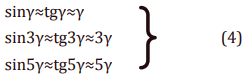The shortest trajectory λ1 consists of two links inclined at angles ±γ. Its length is equal to the wavelength λd de Broglie:

λ1 =λd =2πRb (5)

Length [4 - 7] of longer wavy paths: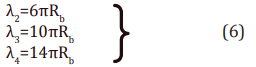The broken wavy trajectories are placed in a uniform coordinate grid with the height and length of the cells k and 1 respectively (Figure 2b).

3. The arithmetic triangle of Pascal was known in ancient India and China . The description of this arithmetic triangle is found in the papers of the poet Omar Khayyam . In , a new first planar analogue of Pascal’s triangle was presented for the case of small angles.

Figure 3 presents various analogs of the Pascal triangle. The first analogue of the Pascal triangle for the case of small angles 4γ is shown in Figure 3a. This analogue was investigated in . In this case, the rays, passing a certain distance (link) of length ≈a, are symmetrically split in two at each iteration at small angles ≈±2γ.

In this paper, we consider the case corr4esponding to our model, but we will assume that the corners γ',3γ',5γ',… are not small that is the expressions (4) for approximate calculations do not hold.

In Figure 3b and Figure 3c two variants of the second analogue of Pascal’s triangle are shown for the case of ordinary angles 4γ'. In this case, the rays, passing a certain distance (link) a, symmetrically split at each iteration in two at the angles of ±2γ' and form parts of a regular polygon.

In the example shown in Figures 3b, c: a≈3,4 [sm], γ'=9°, the radius is inscribed in an regular icosagon circumference r≈10,7 [sm]. After several iterations, a part of the rays overlap each other, as in the Pascal triangle [11, 14] and the first analogue of Pascal’s triangle Figure 3a. Note that the ray patterns in Figure 3a and Figure 3b are similar. It is also not difficult to draw a diagram of rays (at small angles ≈±2γ ) similar to that shown in Figure 3a, but similar to the diagram in Figure 3c.

It is interesting to note that if γ'=30° then in the sequential construction of an analogue of the Pascal triangle (as in Figure 3b), the entire plane will be densely filled with regular hexagons. In other cases (Figure 3b,c), the arrangement of some hexagons will be loose and they will overlap each other.

Such regular hexagons located on a sphere or cylinder can form fullerenes .

In Figure 4, the analog of the Pascal triangle (a) and the ordinary Pascal triangle (b) from  are presented in the form of regular hexagons:

4. Figure 5 shows a wave-like trajectory similar to that shown in Figure 2b, but composed of parts of an icosagon (Figure 3b,c). The wave-like trajectory is placed in an uneven coordinate grid with the height and length of the cells k' and l', respectively. The total length of this trajectory: L=14a (Figure 5); an analogue of λ4 in Figure 2b of the “wave” length: λ'4 = l' 1+l' 2+⋯+l' 14 (Figure 5).

5. Figure 6 for comparison, on the same scale, shows three wavelike curves: 1) a broken wavy line (curve), similar to that shown in Figure 2b, 2) a wave-like curve composed of parts of a regular polygon (Figure 5, 5) sinusoid. The curves are located close to each other, especially when zoomed in along the vertical or at a small angle of inclination of the links from which they are composed.

6. In , we investigated separately the geometric properties of the second shell of an atom. In this case, we considered the sequence shown in Figure 7a. The links located at small angles γ and 3γ are connected in chains, representing broken wavy trajectories. During the first pass (n=l), the ratio K of the number of rays E (weight coefficients) propagating at angles γ and 3γ is:

K(1)=Eγ1(1)+Eγ2(1)/E3γ(1) =2/1 (7)

After a large number of passes (iterations) of n≳100 upon reaching the stationary distribution:

K(≳100)=Eγ1(≳100)+Eγ2(≳100)/E3γ(≳100) =3,0796 (8)

Analytically the result (8) can be obtained by solving a cubic equation using the Cardan formula .

7. Figure 7b shows a similar (diagram in Figure 7a) diagram of wave-like trajectories, but it is composed of rays that are part of a polygonal shape, similar to a regular polygon, as in Figure 5.

For definiteness, we will call the parts of a polygonal shape that form a wave-like trajectory: “a regular polygonal shape”. A regular polygonal shape is similar to a regular polygon, but does not contain an integer number of sides. In our visual constructions we use only parts of this shape. All sides of a regular polygonal shape are equal; you can calculate the radii of the inscribed and circumscribed circles.

In example represented in Figure 7b: a≈1,7 [sm], γ'≈16,8°, the radius r≈2,8 [sm] of circle entered in this regular polygonal shape. The distance of a and the corner γ' were selected for the best presentation for receiving a uniform coordinate grid along horizontal with sizes:

k'1 =k'3 =asinγ';k'2 =asin3γ';l'=(acosγ')/3=(acos3γ')/2 (9)

In this case the relationship is:

2cosγ^'=3cos3γ'. (10)

Or in general:

dcosγ^'=ecos3γ', (11)

Where d and e are some numbers, for example natural.

8. For the case shown in Figure 7b with the first passes, we see separate trajectories. After a large number of passes, the trajectories cover the plane and form a “cloud of trajectories”. Other trajectory schemes can be constructed by specifying other values of the angles γ' and 3γ' and the coefficients d and e for the values cosγ'and cos3γ' in expression (11). The density of this “cloud” in these cases will increase with decreasing angles γ' and 3γ', but after some large number of passes (iterations) it will remain constant (stationary distribution) if the ratio e/d, for example, rational.

Expression (8) is also valid for the case shown in Figure 7b, if we consider not individual rays (links of trajectories) as for the case shown in Figure 7a, but many links (“cloud of links”) at angles γ' and 3γ' after many passes (iterations) located within several passes, for example, three passes, shown in the right part of Figure 7b.

Similarly, based on the consideration of parts of polygonal shapes, one can get the same results of constructions and calculations for other atomic shells consisting of links of longer wavy trajectories, for example, in Figures 2b, 5 and 7b, inclined at different angles [4-7].

In [5,7], we explained the effect of splitting the lines of atomic spectra based on consideration of wavy trajectories inclined at small angles. Perhaps consideration of the trajectories composed of parts of regular polygons will help to more fully explain the complex process of splitting spectra .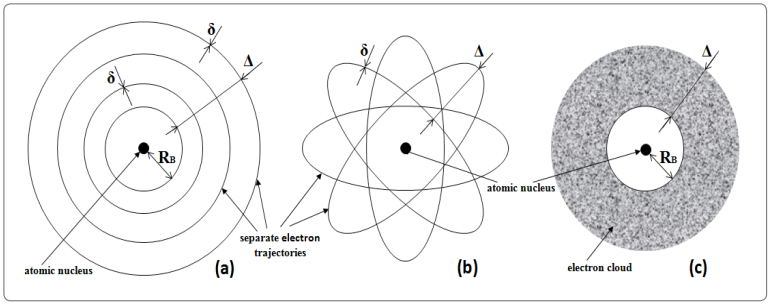Figure 1: Geometric models of the atom consist of lines and geometric shapes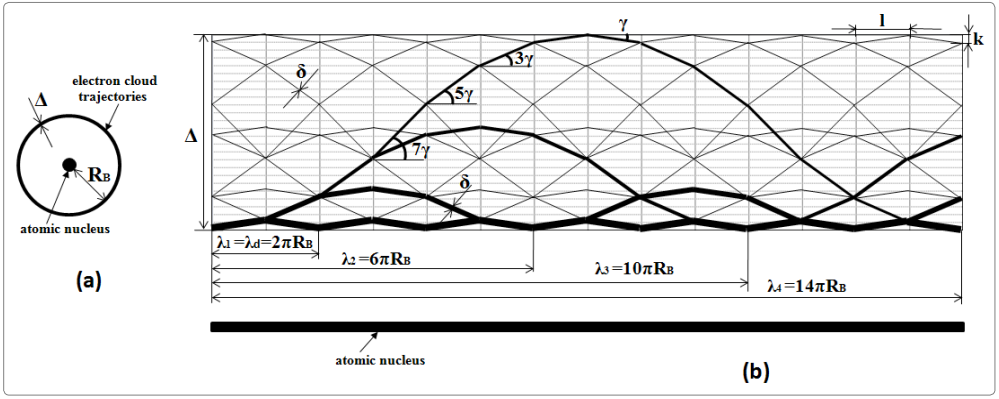Figure 2: New geometric belt nuclear model of the atom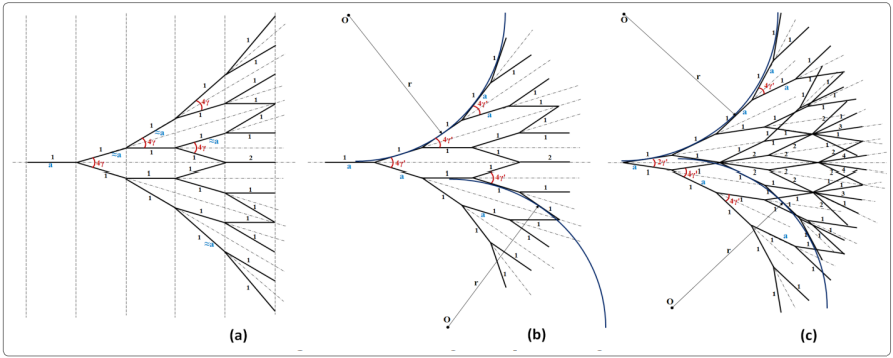Figure 3: Various analogs of the pascal triangle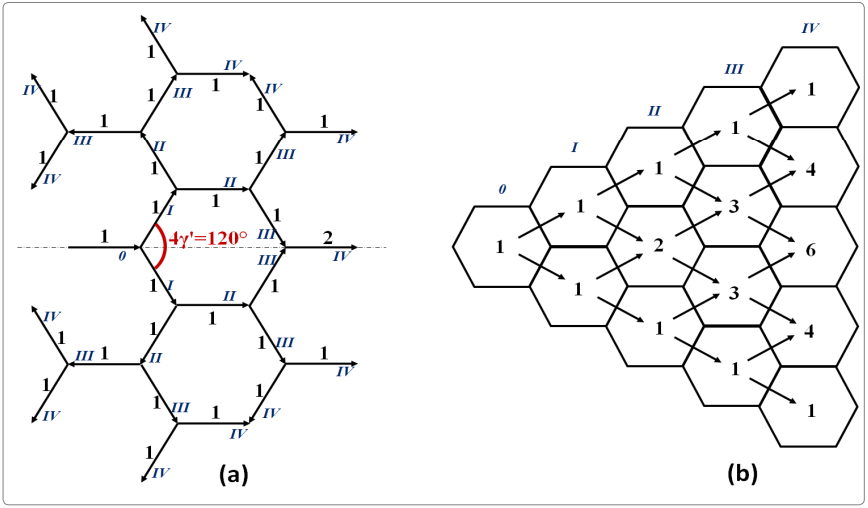Figure 4: (a) The analog of the Pascal triangle, (b) the ordinary Pascal triangle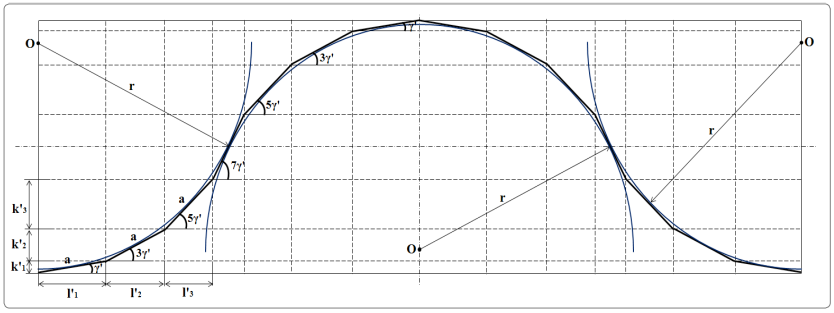Figure 5: A wave-like polygonal trajectory made up of parts of a regular icosagon; parts of the circles inscribed in these figures are shown in blue.